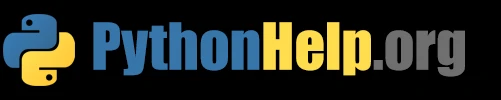# How to draw semi circle with Python

Drawing shapes is an important part of graphics programming, and in this tutorial, we will show you how to draw a semi-circle in Python using the turtle module

Updated March 29, 2023

Hello and welcome to this beginner’s tutorial on how to draw a semi-circle in Python. Drawing shapes is an important part of graphics programming, and in this tutorial, we will show you how to draw a semi-circle in Python using the turtle module.

## Step 1: Import turtle module

To draw shapes in Python, we will use the turtle module. This module provides a simple graphics library that allows you to draw shapes and lines on a canvas. To import the turtle module, simply include the following line at the beginning of your code:

``````import turtle
``````

## Step 2: Draw a semi-circle

To draw a semi-circle using the turtle module, we can use the circle function and set the extent argument to 180 degrees. Here’s an example code that draws a semi-circle with a radius of 100 pixels:

``````import turtle

turtle.speed(0) # Set turtle speed to fastest
turtle.penup() # Lift the pen
turtle.goto(0, -100) # Move turtle to bottom center
turtle.pendown() # Put the pen down
turtle.circle(100, 180) # Draw a semi-circle with radius 100 and 180 degree extent
``````

In this code, we set the turtle speed to the fastest and move the turtle to the bottom center of the canvas. Then we put the pen down and draw a semi-circle with a radius of 100 pixels and a 180 degree extent.

## Step 3: Run the code

After writing the code, simply run it and the semi-circle will be drawn on the canvas. You can customize the size, color, and position of the semi-circle by changing the parameters in the code.

## Conclusion

In this tutorial, we have shown you how to draw a semi-circle in Python using the turtle module. The turtle module provides a simple and easy-to-use graphics library that allows you to draw shapes and lines on a canvas. Remember to experiment with different parameters to create your own unique shapes and designs.

Keep practicing and have fun with Python programming!